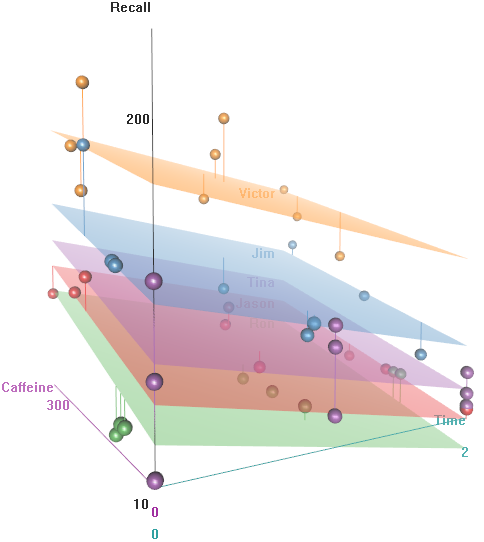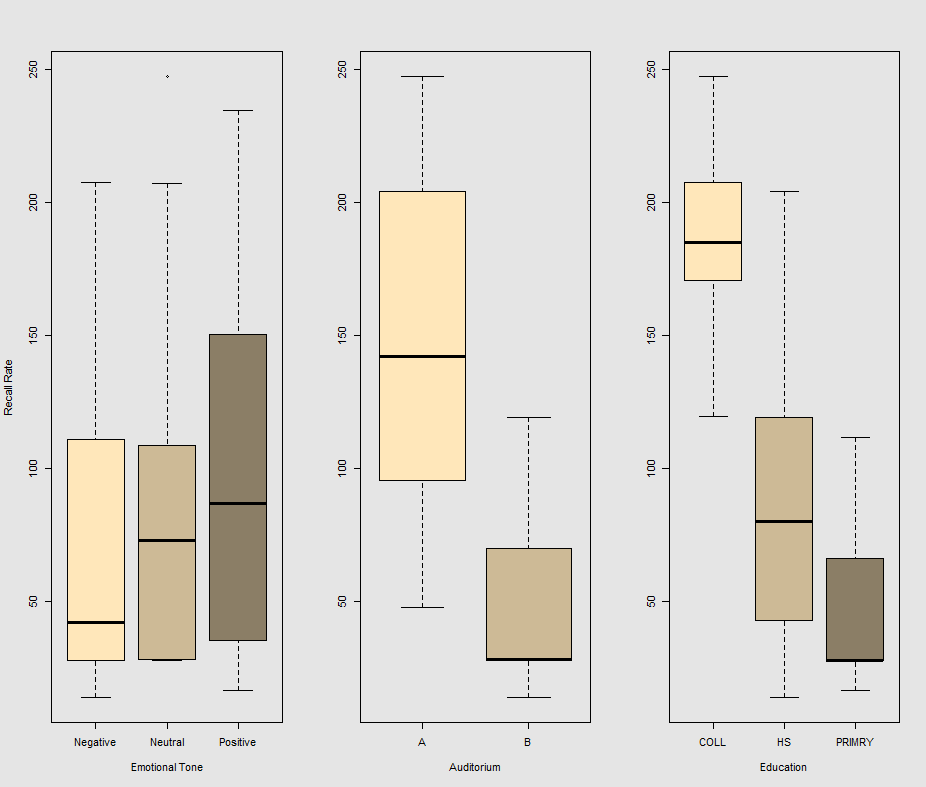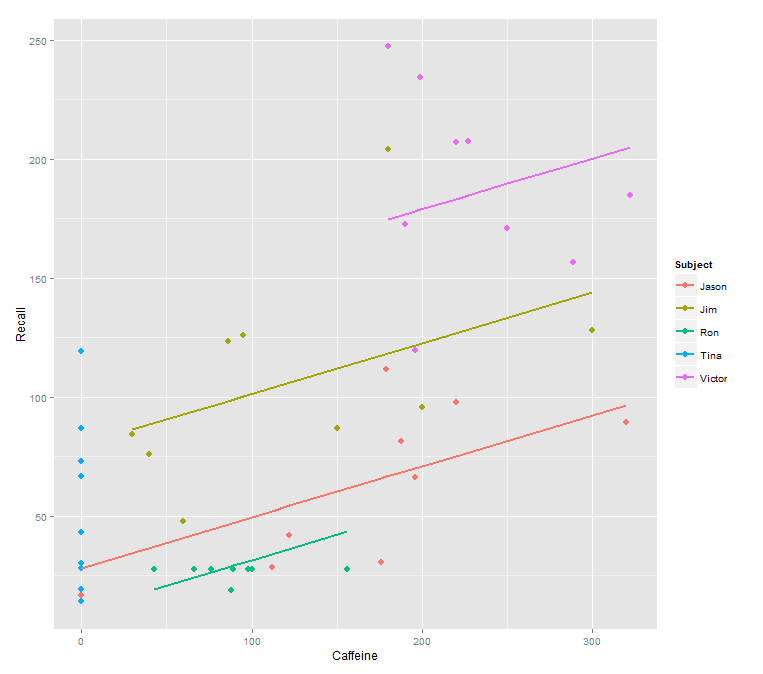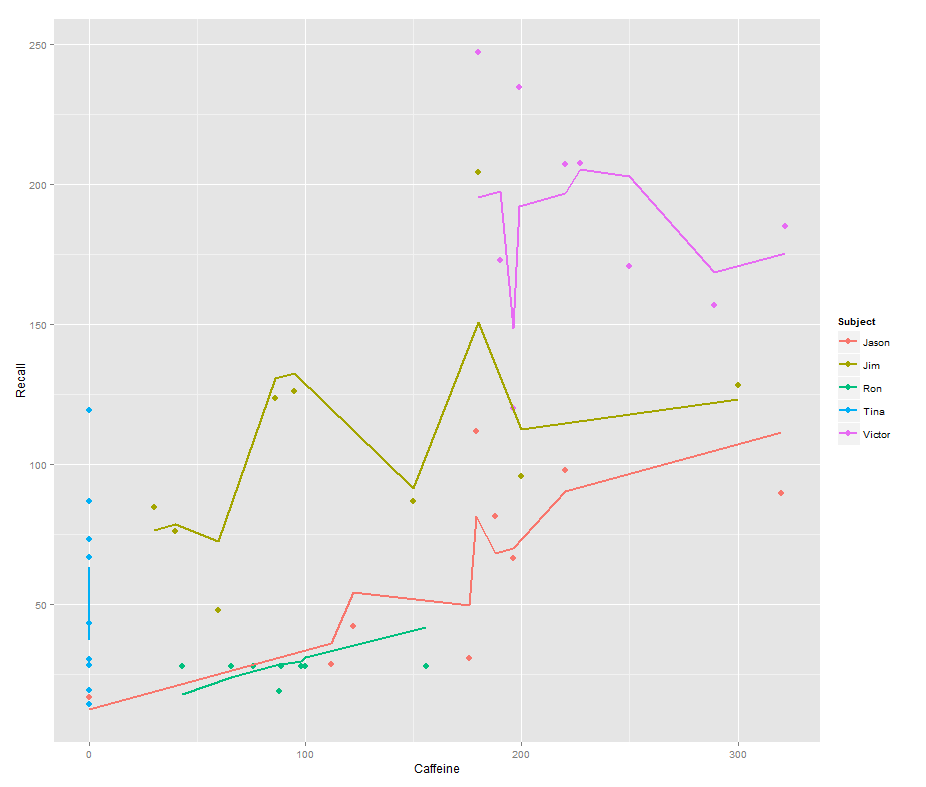### PREDICT FUNCTION IN MIXED EFFECTS MODEL:

I posted this question on CV here.

The problem:

I have read in other posts (a bit old) that predict is not available for mixed effects lmer {lme4} models in [R]. EDIT: Although I know now, thanks to @EdM, that this exists in more recent versions, the question still is unresolved in terms of the actual algebra from $$intercepts$$ and $$slopes$$ -> $$predicted$$ values.

However, in trying to get a more plastic sense of linear effects, I came across a situation where the predict call did seem to incorporate the random effects in the model, yielding a plausible output.

Background:

I’m working with an extremely massaged toy dataset. For the sake of intellectual honesty, I think I got the idea of the set from a Princeton on-line class, but any similarity to the original is at this point coincidental. In unsuccessfully looking for the original, I did come across this that can serve as indirect credit and much more.

If anybody is so inclined to take a look directly it can be retrieved directly:

require(gsheet)
format ='csv'))

Subject Auditorium Education Time  Emotion Caffeine Recall
1     Jim          A        HS    0 Negative       95 125.80
2     Jim          A        HS    0  Neutral       86 123.60
3     Jim          A        HS    0 Positive      180 204.00

So we have some repeated observations (Time) of a continuous measurement of the Recall rate of some words, and several $$\small DV$$’s, including random effects (Auditorium where the test took place; Subject name); and explanatory or fixed effects, such as Education, Emotion (the emotional connotation of the word to remember), or $$\small mgs.$$ of Caffeine ingested prior to the test.

The idea is that it’s easy to remember for hyper-caffeinated wired subjects, but the ability decreases over time, perhaps due to tiredness. Words with negative connotation are more difficult to remember. Education has an effect that is intuitive, and “surprisingly” the Auditorium also plays a role (perhaps one was more noisy, or less comfortable). Here’re a couple of exploratory plots:I know… so cool… Have you noticed that Tina doesn’t drink coffee? How can anybody spend the night on CV, and be able to function in the morning without coffee?The issue:

When fitting lines on the data cloud for the call:

fit1 <- lmer(Recall ~ (1|Subject) + Caffeine, data = data)

I get this plot:with the following code (notice the call for $$\small predict(fit1)$$) in it:

library(ggplot2)
p <- ggplot(data, aes(x = Caffeine, y = Recall, colour = Subject)) +
geom_point(size=3) +
geom_line(aes(y = predict(fit1)),size=1)
print(p)

while the following model:

fit2 <- lmer(Recall ~ (1|Subject/Time) + Caffeine, data = data)

incorporating Time and a parallel code gets a surprising plot:

p <- ggplot(data, aes(x = Caffeine, y = Recall, colour = Subject)) +
geom_point(size=3) +
geom_line(aes(y = predict(fit2)),size=1)
print(p)The question:

How does the predict function operate in this lmer model? Evidently it’s taking into consideration the Time variable, resulting in a much tighter fit, and the zig-zagging that is trying to display this third dimension of Time portrayed in the first plot.

If I call predict(fit2) I get 132.45609 for the first entry, which corresponds to the first point: Subject = Jim, Auditorium = A, Education = HS, Time = 0, Emotion = Negative, Caffeine = 95 and Recall = 125.8

The coefficients for fit2 are:

$Time:Subject (Intercept) Caffeine 0:Jason 75.03040 0.2116271 0:Jim 94.96442 0.2116271 0:Ron 58.72037 0.2116271 0:Tina 70.81225 0.2116271 0:Victor 86.31101 0.2116271 1:Jason 59.85016 0.2116271 1:Jim 52.65793 0.2116271 1:Ron 57.48987 0.2116271 1:Tina 68.43393 0.2116271 1:Victor 79.18386 0.2116271 2:Jason 43.71483 0.2116271 2:Jim 42.08250 0.2116271 2:Ron 58.44521 0.2116271 2:Tina 44.73748 0.2116271 2:Victor 36.33979 0.2116271$Subject
(Intercept)  Caffeine
Jason     30.40435 0.2116271
Jim       79.30537 0.2116271
Ron       13.06175 0.2116271
Tina      54.12216 0.2116271
Victor   132.69770 0.2116271

My best bet was 94.96442 + 0.2116271 * 95 = 115.0689945… Not too far off, but wrong. What is the formula to get instead to 132.45609?

It’s easy to get confused by the presentation of coefficients when you call coef(fit2). Look at the summary of fit2:

> summary(fit2)
Linear mixed model fit by REML ['lmerMod']
Formula: Recall ~ (1 | Subject/Time) + Caffeine
Data: data
REML criterion at convergence: 444.5

Scaled residuals:
Min       1Q   Median       3Q      Max
-1.88657 -0.46382 -0.06054  0.31430  2.16244

Random effects:
Groups       Name        Variance Std.Dev.
Time:Subject (Intercept)  558.4   23.63
Subject      (Intercept) 2458.0   49.58
Residual                  675.0   25.98
Number of obs: 45, groups:  Time:Subject, 15; Subject, 5

Fixed effects:
Estimate Std. Error t value
(Intercept) 61.91827   25.04930   2.472
Caffeine     0.21163    0.07439   2.845

Correlation of Fixed Effects:
(Intr)
Caffeine -0.365

There is an overall intercept of 61.92 for the model, with a caffeine coefficient of 0.212. So for caffeine = 95 you predict an average 82.06 recall.

Instead of using coef, use ranef to get the difference of each random-effect intercept from the mean intercept at the next higher level of nesting:

> ranef(fit2)
$Time:Subject (Intercept) 0:Jason 13.112130 0:Jim 33.046151 0:Ron -3.197895 0:Tina 8.893985 0:Victor 24.392738 1:Jason -2.068105 1:Jim -9.260334 1:Ron -4.428399 1:Tina 6.515667 1:Victor 17.265589 2:Jason -18.203436 2:Jim -19.835771 2:Ron -3.473053 2:Tina -17.180791 2:Victor -25.578477 $Subject
(Intercept)
Jason   -31.513915
Jim      17.387103
Ron     -48.856516
Tina     -7.796104
Victor   70.779432

The values for Jim at Time=0 will differ from that average value of 82.06 by the sum of both his Subject and his Time:Subject coefficients:

$82.06+17.39+33.04=132.49$

which I think is within rounding error of 132.46.

The intercept values returned by coef seem to represent the overall intercept plus the Subject or Time:Subject specific differences, so it’s harder to work with those; if you tried to do the above calculation with the coef values you would be double-counting the overall intercept.